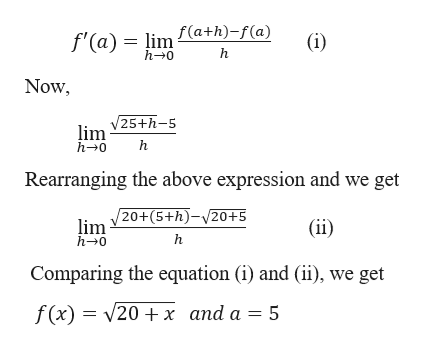the limit         limh→0  (√(25+h) - 5)/hrepresents the derivative of some function f(x) at some number a. Find f and a.

Question

the limit

limh→0  (√(25+h) - 5)/h

represents the derivative of some function f(x) at some number a. Find f and a.

Step 1

We know th...help_outlineImage Transcriptionclosef'(a) = lim £(a+h) -f(a) h 0 (i) h Now lim V25+h-5 h-0 Rearranging the above expression and we get lim V20+(5+h)-v20+5 (ii) h-0 Comparing the equation (i) and (ii), we get f(x)20x and a = 5 fullscreen

Want to see the full answer?

See Solution

Want to see this answer and more?

Our solutions are written by experts, many with advanced degrees, and available 24/7

See Solution
Tagged in

Derivative